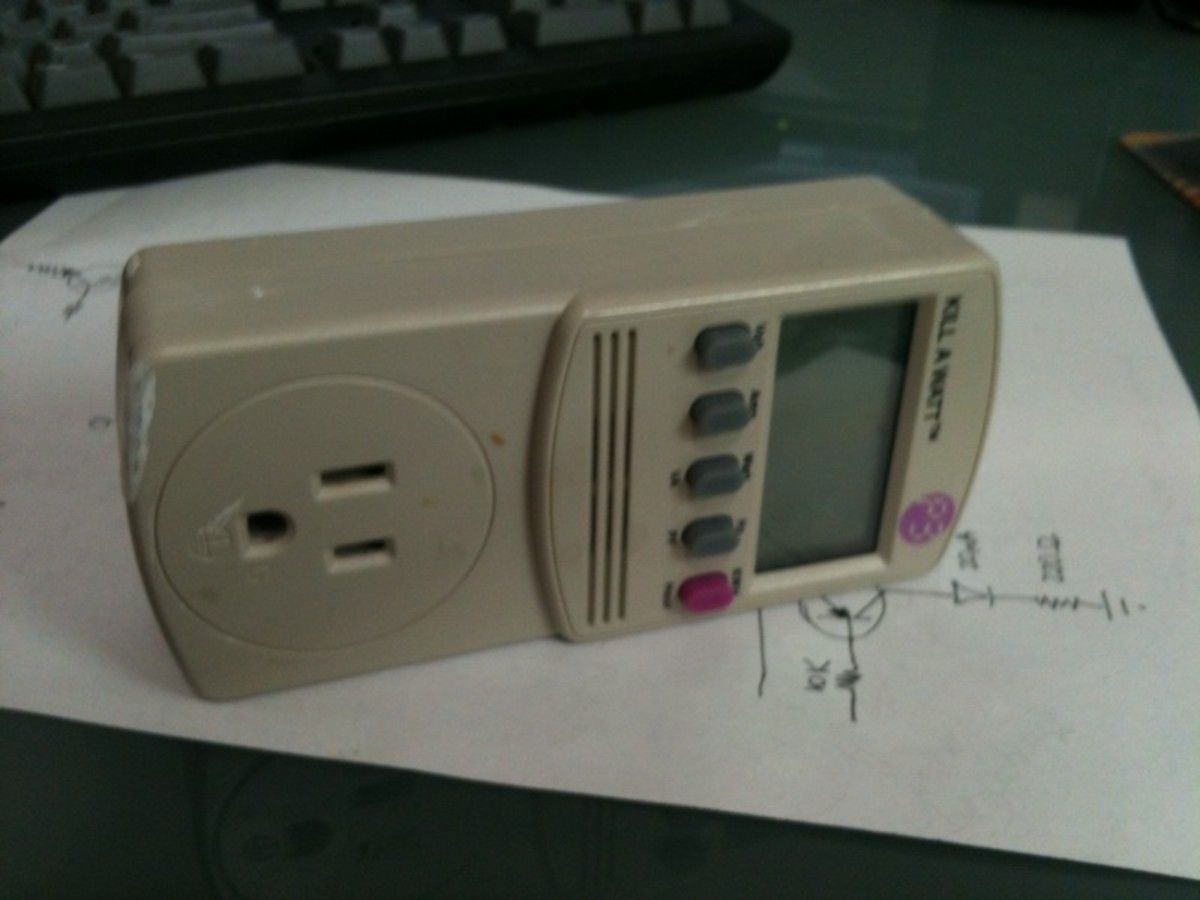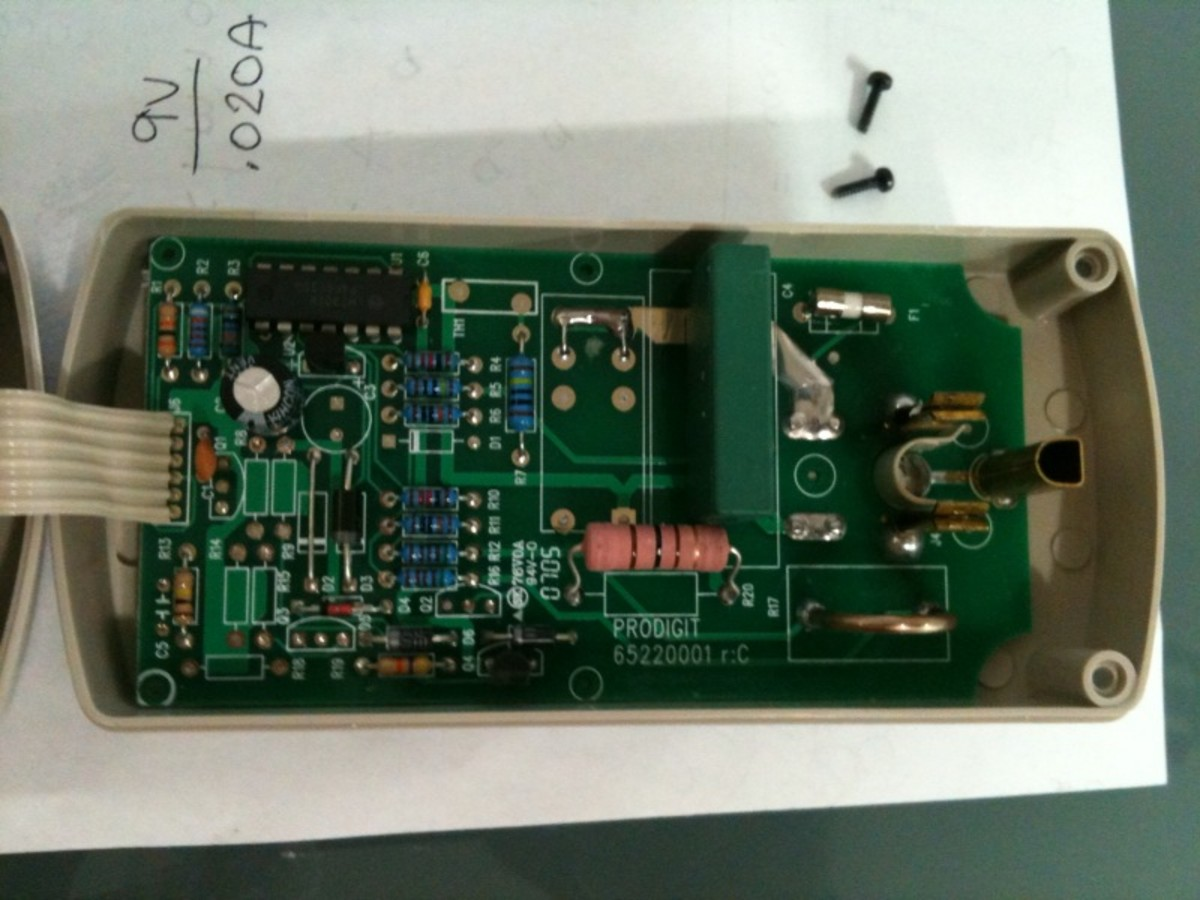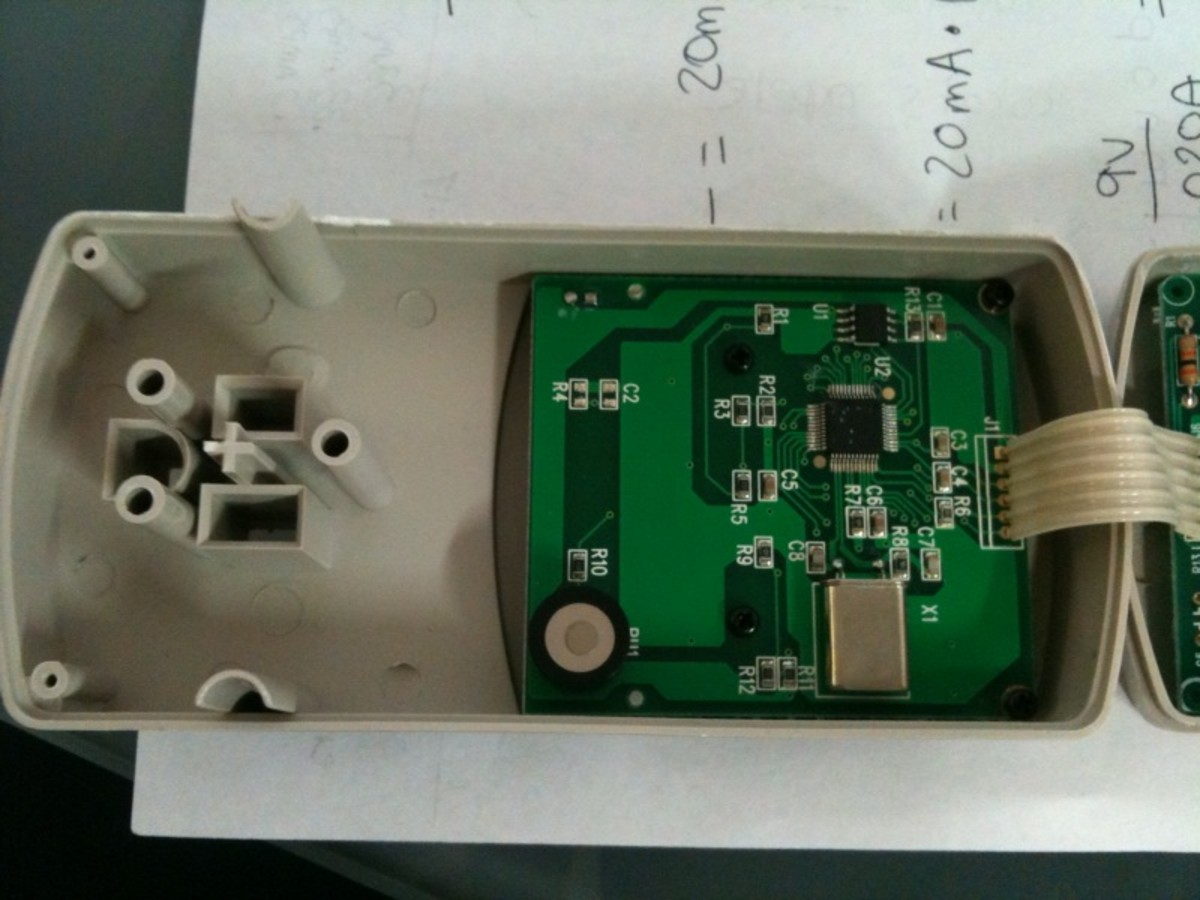# Make Your Own Wattmeter With A Microcontroller

## Watt Meter PictureA typical commercially available watt meterwatt meter exampleInside a watt meter. This is the current sense board which converts the current into a voltage which can be interpreted by a microcontroller.The micrcontroller board inside a watt meter. This circuit performs the logical steps to convert amps and volts to power and displays it on an LCD.

A watt meter, like the Kill A Watt pictured, plugs into the wall and records power usage statistics about the devices which are plugged into it. The exact details of how this is accomplished may differ, since there are many ways one may consider to design and implement such a device, but the basic idea is the same. Opening the Kill A Watt model reveals some of the secrets it employs to make a power measurement.

Electricity is passed from the wall outlet through a fuse and through a current sense resistor. Attached to the current sense resistor is a small circuit which amplifies the very small current coming from the sense resistor into a voltage which can be read by a microcontroller. The microcontroller converts this voltage using an analog to digital converter (ADC) and also takes into consideration the voltage, which is roughly 120 volts, and ultimately computes the power.

At least that is what the Kill A Watt appears to be doing.However, this page is not devoted to this particular watt meter, instead we're here to talk about how to build a watt meter, using our own design, from scratch!

The schematic above revolves around the MCP601 op amp chip wired to function as a high gain amplifier. The light bulb, in the circuit, represents a household type appliance which is the device under consideration by the circuit. As the household's electricity runs through the circuit it powers the light bulb and goes through the very low resistance current sense resistor. The voltage across the low resistance resistor will be very close to zero, but not quite. In fact, the voltage will be proportional to the amount of current flowing through it, but always a small and hard to detect amount. That's where the op amp comes in. It multiplies the small voltage present on the resistor and sends it to an ADC (analog to digital converter) on a microcontroller for reading. The microcontroller takes a voltage from 0-Vcc and converts it to a digital value between 0 and 255 (for an 8 bit conversion) or 0-1023 (for a 10 bit conversion). For this reason, to get a full scale reading requires some sort of scaling circuit (not pictured).

The point of scaling is to determine beforehand what the range of operation the watt meter must accept. The Kill A Watt model I have is rated for up to 15 amps, which is the max rating for the outlet anyway so it's probably enough for our design too. So, we tune the op amp circuit, by adjusting resistor values (a potentiometer works well for this) to enable a certain voltage sweep when reading appliance currents from 0 to 15 amps.

Power is equal to voltage times current, so in other words, watts = volts * amps. Now for many designers, 120 volts may suffice for the pre-programmed calculation for the microcontroller to use. Unfortunately, if more accuracy is needed a voltage sensor will also need to be used to measure the incoming voltage on the AC circuit. Voltage from the power company is not very precise and tends to experience slow fluctuations over the course of the day. When the voltage is lower, appliances will need to draw more current to get the same amount of power. If the watt meter doesn't know that the voltage has changed it will think that suddenly the appliance is more power hungry, which isn't actually the case. Now, one more problem, the main electricity in the home is AC, and about 60 Hz (but not exactly) , in the United States. For more precise calculations, one may even consider measuring the frequency on the line, against a high precision crystal oscillator or atomic clock!

Consider the fact that the voltage on the line is alternating like a sine wave. This means that at any given instantaneous moment, its voltage could be anything from 0 volts to the peak voltage of the sine wave. So why do we say it is 120 volts? It is because it is very convenient to think of AC voltage in terms of averages rather than instantaneous values. 120 volts is the average value of the sine wave of the electricity or its RMS value (root mean square). This means that the peak value is about 170 volts from 0 on the positive side and 170 volts from zero on the negative side. If you take 170 volts multiplied by the sqrt(2)/2, that would give you 120 volts! The difference in way AC current and consequently AC power can be measured may lead to some ambiguities. RMS is almost always implied if not explicitly mentioned.

Back to digital electronics... Before the AC voltage can be worked with, it must be converted to a lower DC form. Since DC cannot take on multiple ambiguous values, you must decide which measurement of the AC you're taking before converting to DC. If the RMS value is to be used, which is recommended, then the DC value converted from the AC will have to be proportional to the 120 volts RMS value. Of course, 120 volts is too high for any microcontroller to handle, so it will need to be scaled down to an appropriately low level. Also, once again, just as for the current readings, the designer must decide the tolerable range of voltage that the device can adjust to work with. One approach might be to accept any RMS voltages from 100 to 150 volts, this would work in all conceivable cases in the US for standard household 120 volt circuits. This 50 volt window (100-150 volts) must be converted to a microcontroller safe value of, for example, 0 to 3.3 volts. Assuming proportionality, using this scheme, the microcontroller would read a value on the ADC of about 1.32 volts when the original AC input was 120 volts. The microcontroller would then register this digitally as 410 (in a 10 bit system).

I'm skimming over many of the fine details, but that's the specifics that would make the design your own! This article is intended as a strategy for how to go about designing such a device. Anyway, a little more about the microcontroller, which is, after all, the brain of the system.

The microcontroller has to have at least two ADCs to make the current and voltage measurements. Internally, the microcontroller represents these readings as a digital value from between 0 and 1023 (for the 10 bit ADC). It's important to remember the conversion factors to convert these digital seemingly arbitrary values back to the voltages and amperages they represent. In my previous example, a reading of 410 resulted from a voltage of 120 volts so the conversion factor would simply be 0.292. Multiplying this factor by the digital value stored by the ADC will result in the actual voltage. The current measurement would likely have a different factor, but the same process would be used, and when the values are de-mystified, they would be multiplied together, at run-time, by the microcontroller, and the result would be the power (in watts) that the particular external appliance was consuming.

Scroll to Continue

Now... the fun part. The power meter's job is to display this final piece of information (watts) in a human usable form. The end product may have button's which connect to the microcontroller's I/O pins and be programmed to change modes depending on which buttons are pressed. For my own design, I chose to output the information using a multiplexed multidigit LED display. But LCD, would have been a more energy conservative approach. Many microcontrollers, like the PIC18F2525, for example, have an internal EEPROM memory bank, which is non-volatile, and can be used to store periodic power usages over the course of a few days to help the user evaluate the long term power usages of their household devices. Data logging features are a must have for any serious power watt meter.

Prachi on October 14, 2013:

What if I wanted to measure DC current and voltage and my load is a DC light bulb?

ckimani from Kenya on July 10, 2012:

thanks a lot am doing a project on obtaining the power of a load and this info will.be of help

npolynomial (author) on June 27, 2012:

Hey Tim,

In simple direct current circuits power would be equal to volts * amps.

In a single phase AC circuit, one can measure the effective average voltage or current (which assuming a sinusoid wave) would involve measuring the peak instantaneous voltage (or current) and multiplying it by the (square root of 2 ) / 2, which would yield approx. 0.70711. Multiplying the peak by this, would yield the average value. The trick then with this circuit is to measure the instantaneous voltages and currents at a high enough sampling rate to determine accurate average values. By this method, whether the supply was sinusoidal, square wave, triangle, white noise, etc... the circuit will still accurately determine the total power transmission.

Tim on June 12, 2012:

Er, last time I checked, P = V*I*powerfactor

DC on January 21, 2012:

Very good post, thank you!

Final year project presentation on May 12, 2011:

I was lucky to find this hub , searching for a long time...thanks..